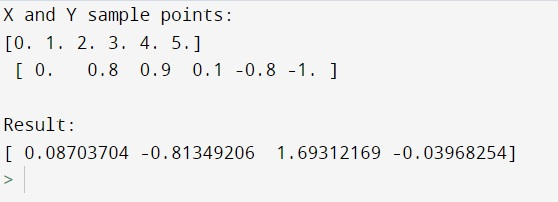# numpy.polyfit() Method with Example

Python | numpy.polyfit(): Learn about the numpy.polyfit() method, its usages and example.
Submitted by Pranit Sharma, on February 27, 2023

NumPy is an abbreviated form of Numerical Python. It is used for different types of scientific operations in python. Numpy is a vast library in python which is used for almost every kind of scientific or mathematical operation. It is itself an array which is a collection of various methods and functions for processing the arrays.

## numpy.polyfit() Method

The numpy.polyfit() method is used for finding the best fitting curve to a given set of points by minimizing the sum of squares, which means that this function helps us by finding the least square polynomial fit.

A mathematical expression generally composed of more than one variable where the degree of the variable is usually a whole number is said to be a polynomial.

If there is a polynomial of degree k to points (x,y), it fits a polynomial p(x) = p * x**k + ... + p[k]. It returns a vector of coefficients p that minimizes the squared error in the order k, k-1, … 0.

Syntax:

```numpy.polyfit(x, y, deg, rcond=None, full=False, w=None, cov=False)
```

Parameter(s):

• x: x-coordinates of sample points.
• Y: y coordinates of sample points.
• deg: degree of polynomial fit
• rcond: relative condition number of the fit.

Let us understand with the help of an example,

## Python code to demonstrate the example of numpy.polyfit() method

```import numpy as np

# Creating points for x
x = np.array([0.0, 1.0, 2.0, 3.0,  4.0,  5.0])

# Creating points for y
y = np.array([0.0, 0.8, 0.9, 0.1, -0.8, -1.0])

# Display x and y
print("X and Y sample points:\n",x,"\n",y,"\n")

# Using numpy.polyfit
res = np.polyfit(x,y,3)

# Display result
print("Result:\n",res)
```

Output:Languages: » C » C++ » C++ STL » Java » Data Structure » C#.Net » Android » Kotlin » SQL
Web Technologies: » PHP » Python » JavaScript » CSS » Ajax » Node.js » Web programming/HTML
Solved programs: » C » C++ » DS » Java » C#
Aptitude que. & ans.: » C » C++ » Java » DBMS
Interview que. & ans.: » C » Embedded C » Java » SEO » HR
CS Subjects: » CS Basics » O.S. » Networks » DBMS » Embedded Systems » Cloud Computing
» Machine learning » CS Organizations » Linux » DOS
More: » Articles » Puzzles » News/Updates